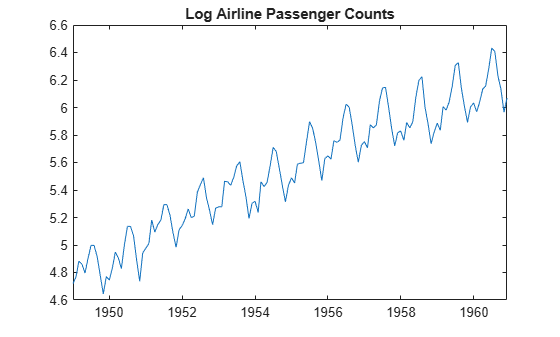Documentation

## Moving Average Trend Estimation

This example shows how to estimate long-term trend using a symmetric moving average function. This is a convolution that you can implement using `conv`. The time series is monthly international airline passenger counts from 1949 to 1960.

Load the airline data set (`Data_Airline`).

```load(fullfile(matlabroot,'examples','econ','Data_Airline.mat')) y = log(Data); T = length(y); figure plot(y) h = gca; h.XLim = [0,T]; h.XTick = [1:12:T]; h.XTickLabel = datestr(dates(1:12:T),10); title 'Log Airline Passenger Counts'; hold on```The data shows a linear trend and a seasonal component with periodicity 12.

The periodicity of the data is monthly, so a 13-term moving average is a reasonable choice for estimating the long-term trend. Use weight 1/24 for the first and last terms, and weight 1/12 for the interior terms. Add the moving average trend estimate to the observed time series plot.

```wts = [1/24;repmat(1/12,11,1);1/24]; yS = conv(y,wts,'valid'); h = plot(7:T-6,yS,'r','LineWidth',2); legend(h,'13-Term Moving Average') hold off```When you use the shape parameter `'valid'` in the call to `conv`, observations at the beginning and end of the series are lost. Here, the moving average has window length 13, so the first and last 6 observations do not have smoothed values.